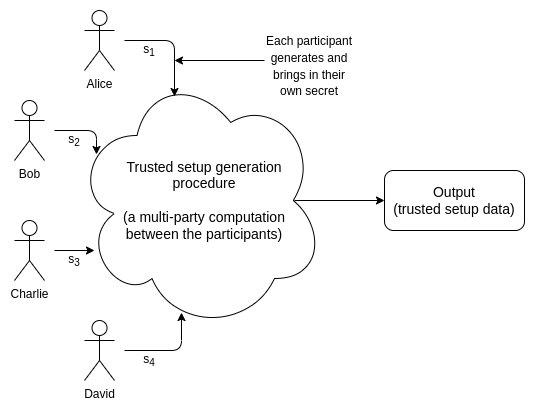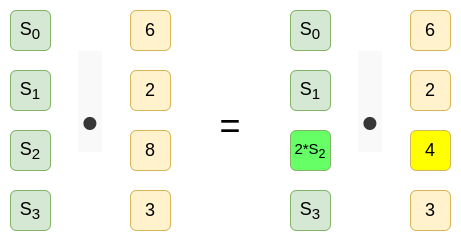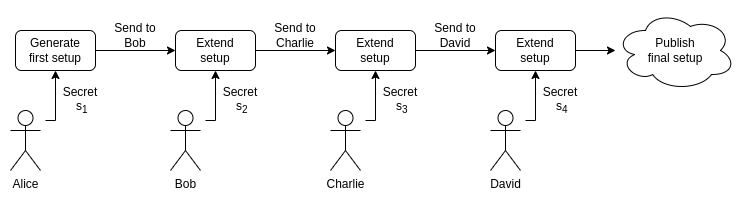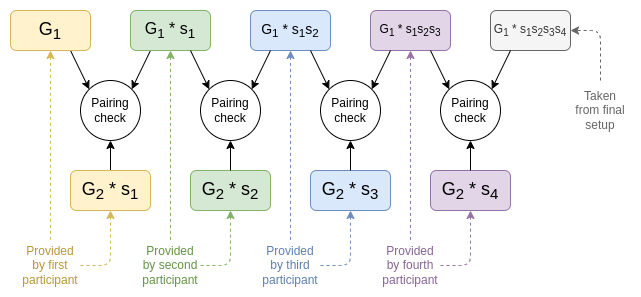# Vitalik：受信任初始化的原理是什么?

KZG 受信任初始化对 Danksharding 的实现起着至关重要的作用，本文介绍了受信任初始化的运作原理以及其验证过程。

《Vitalik: Proto-Danksharding FAQ》

《大幅简化的新分片设计》

《分片 + 数据可用性采样》## Powers-of-tau 初始化是怎样的？

powers-of-tao初始化由两系列的椭圆曲线点组成，如下所示：

[$G{1}$, $G{1}s$,$G_{1}s^{2}$…$G{1}*s^{n{1} -1} ]$

[$G{2}$,$G{2}s$ , $G_{2}s^2$…$G{2} *s^{n{2}−1}$]

$G{1}$ 和 $G{2}$是两个椭圆曲线群的标准生成元；在 BLS12-381 中，$G{1}$占用 48 字节（压缩形式）， $G{2}$占用 96 字节。$n{1}$, $n{2}$分别是初始化输出的$G{1}$, $G{2}$生成点列的长度。一些协议要求 $n{2}=2$，另一些协议要求 $n{1}$和 $n{2}$的值都较大，一些协议属于中间情形（例如，当前的以太坊数据可用性采样方案要求 $n{1}=4096$ 和 $n_{2}=16$）。$s$ 是用于生成点列的秘密值，使用后需要销毁。

## 直观地说，受信任初始化提供了什么价值？## 多方受信任初始化如何运作？$\left [ G{1},G{1}\left ( st \right ) ,G{1}\left ( st^{2} \right ) …G{1} \left ( st^{n{1}-1} \right ) \right ]$ $\left [ G{2},G_{2}\left ( st \right ) ,G{2}*\left ( st^{2} \right ) …G{2} *\left ( st^{n_{2}-1} \right ) \right ]$ 也就是说，您已经创建了一个秘密值 $s∗t$ 相对应的有效初始化输出！你永远不会把你的秘密值 $t$ 给到之前的参与者，同时之前的参与者也不会把他们的秘密值 $s$ 给到你。只要任意一个参与者是诚实的，并且没有透露他那部分的秘密值，那么组合起来的秘密值就不会被泄露。特别地，有限域具有这样的性质：如果你知道 $s$ 但不知道 $t$，并且 $t$ 是被安全随机地生成的，那么你对 $s∗t$ 的值一无所知！

## 验证受信任初始化$$s{1}$$ 是第一个参与者的秘密值，$$s{2}$$ 是第二个参与者的秘密值，以此类推。在每个步骤中的配对检查验证了每个步骤的初始化输出确实源自前一步骤初始化输出以及参与者在当前步骤中已知的新秘密值的组合。

（译者注：配对的特性 $e(G{1}*s{1}, G{2}*s{2}) = e(G{1}*s{1}s{2}, G{2})$）

## 受信任初始化的未来

Powers-of-tau 并不是唯一的受信任初始化方案。其他一些（实际上或潜在）值得注意的受信任初始化方案包括：

• 旧版的 ZK-SNARK 协议中使用的更为复杂的初始化方案（例如，参见此处)有时仍会被使用（特别地，Groth16)，因为它验证成本会比 PLONK 更低。

• 一些密码协议（例如，DARK) 依赖于隐阶群，群中元素不知道进行多少次乘法运算才能得到零元素。目前存在着完全无信任的版本（请参阅：class groups)，但目前为止，最高效的版本使用的是 RSA 群（$x$的幂 mod $n=pq$，其中 $p$，$q$未知）。遵循 1-of-n 信任假设的受信任初始化方案是可能的，但实现起来非常复杂。

• 如果/当不可区分混淆变得可行时，许多依赖于它的协议将会涉及：某人创建和发布一个混淆程序，该程序使用内部的隐藏秘密来执行某些操作。这就是受信任初始化流程：创建者需要持有秘密值来创建程序，而且之后需要把秘密值删除。

ECN的翻译工作旨在为中国以太坊社区传递优质资讯和学习资源，文章版权归原作者所有，转载须注明原文出处以及ethereum.cn，若需长期转载，请联系eth@ecn.co进行授权。

• 发表于 2022-08-01 20:42
• 阅读 ( 59 )
• 学分 ( 0 )
• 分类：以太坊